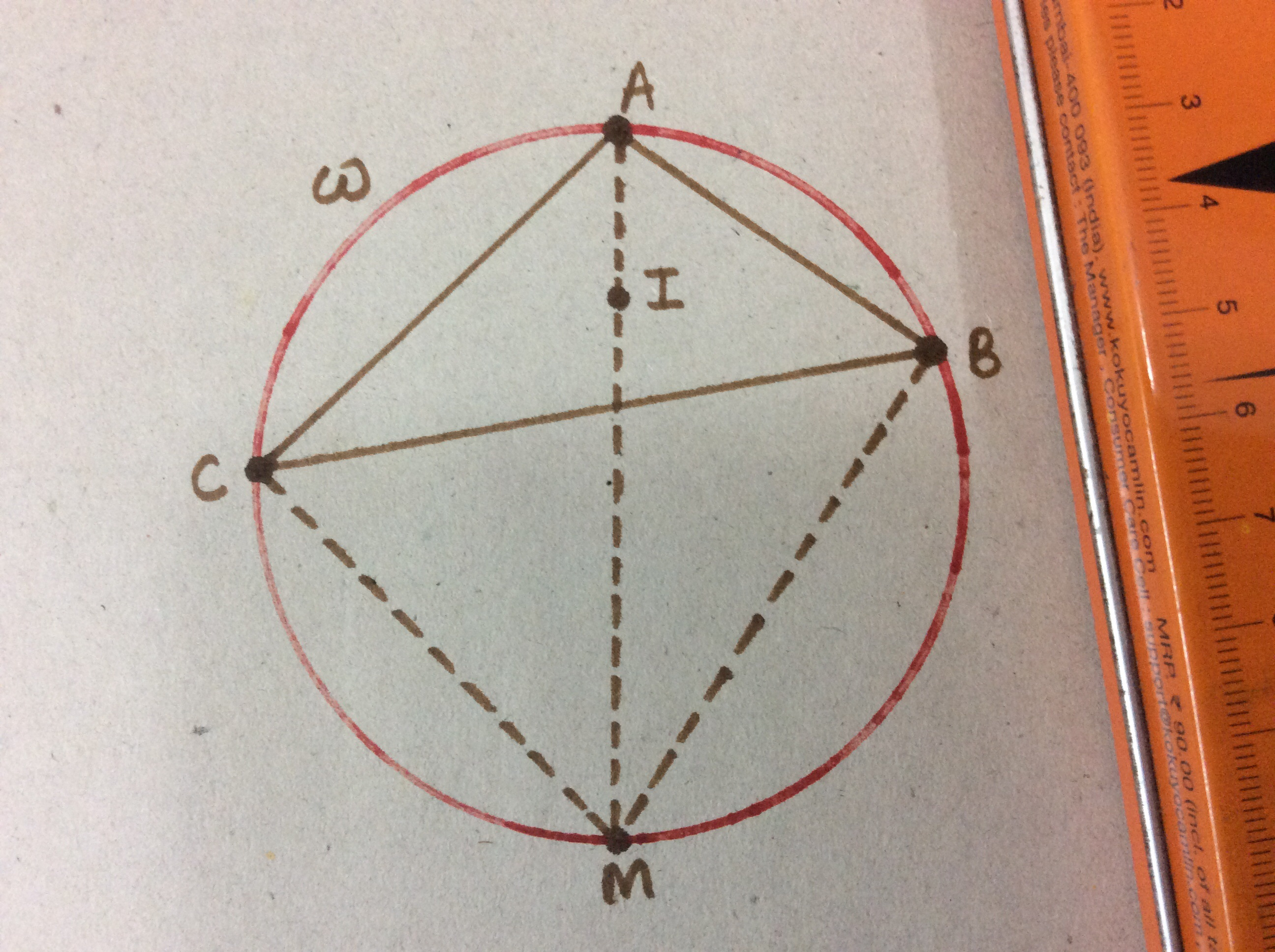# Elegance is the key - Part III

Geometry Level 3Consider a $\Delta ABC$ with incentre $I$ and circumcircle $\omega$. Extend $\overline {AI}$ such that it meets $\omega$ at $M$ on the other side, as shown in the figure. Given that $\overline {MI} = 6$ units, find $\overline {MB} + \overline {MC}$.

×

Problem Loading...

Note Loading...

Set Loading...